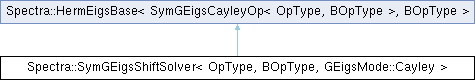Spectra  1.0.1 Header-only C++ Library for Large Scale Eigenvalue Problems
Spectra::SymGEigsShiftSolver< OpType, BOpType, GEigsMode::Cayley > Class Template Reference

#include <Spectra/SymGEigsShiftSolver.h>

Inheritance diagram for Spectra::SymGEigsShiftSolver< OpType, BOpType, GEigsMode::Cayley >:## Public Member Functions

SymGEigsShiftSolver (OpType &op, BOpType &Bop, Index nev, Index ncv, const Scalar &sigma)Public Member Functions inherited from Spectra::SymEigsBase< SymGEigsCayleyOp< OpType, BOpType >, BOpType >
void init (const Scalar *init_resid)

void init ()

Index compute (SortRule selection=SortRule::LargestMagn, Index maxit=1000, Scalar tol=1e-10, SortRule sorting=SortRule::LargestAlge)

CompInfo info () const

Index num_iterations () const

Index num_operations () const

Vector eigenvalues () const

virtual Matrix eigenvectors (Index nvec) const

virtual Matrix eigenvectors () const

## Detailed Description

### template<typename OpType, typename BOpType> class Spectra::SymGEigsShiftSolver< OpType, BOpType, GEigsMode::Cayley >

This class implements the generalized eigen solver for real symmetric matrices using the Cayley spectral transformation. The original problem is to solve $$Ax=\lambda Bx$$, where $$A$$ is symmetric and $$B$$ is positive definite. The transformed problem is $$(A-\sigma B)^{-1}(A+\sigma B)x=\nu x$$, where $$\nu=(\lambda+\sigma)/(\lambda-\sigma)$$, and $$\sigma$$ is a user-specified shift.

This solver requires two matrix operation objects: one to compute $$y=(A-\sigma B)^{-1}x$$ for any vector $$v$$, and one for the matrix multiplication $$Bv$$.

If $$A$$ and $$B$$ are stored as Eigen matrices, then the first operation object can be created using the SymShiftInvert class, and the second one can be created using the DenseSymMatProd or SparseSymMatProd classes. If the users need to define their own operation classes, then they should implement all the public member functions as in those built-in classes.

Template Parameters
 OpType The type of the first operation object. Users could either use the wrapper class SymShiftInvert, or define their own that implements the type definition Scalar and all the public member functions as in SymShiftInvert. BOpType The name of the matrix operation class for $$B$$. Users could either use the wrapper classes such as DenseSymMatProd and SparseSymMatProd, or define their own that implements all the public member functions as in DenseSymMatProd. Mode Mode of the generalized eigen solver. In this solver it is Spectra::GEigsMode::Cayley.

Definition at line 399 of file SymGEigsShiftSolver.h.

## ◆ SymGEigsShiftSolver()

template<typename OpType , typename BOpType >
 Spectra::SymGEigsShiftSolver< OpType, BOpType, GEigsMode::Cayley >::SymGEigsShiftSolver ( OpType & op, BOpType & Bop, Index nev, Index ncv, const Scalar & sigma )
inline

Constructor to create a solver object.

Parameters
 op The matrix operation object that computes $$y=(A-\sigma B)^{-1}v$$ for any vector $$v$$. Users could either create the object from the wrapper class SymShiftInvert, or define their own that implements all the public members as in SymShiftInvert. Bop The $$B$$ matrix operation object that implements the matrix-vector multiplication $$Bv$$. Users could either create the object from the wrapper classes such as DenseSymMatProd and SparseSymMatProd, or define their own that implements all the public member functions as in DenseSymMatProd. $$B$$ needs to be positive definite. nev Number of eigenvalues requested. This should satisfy $$1\le nev \le n-1$$, where $$n$$ is the size of matrix. ncv Parameter that controls the convergence speed of the algorithm. Typically a larger ncv means faster convergence, but it may also result in greater memory use and more matrix operations in each iteration. This parameter must satisfy $$nev < ncv \le n$$, and is advised to take $$ncv \ge 2\cdot nev$$. sigma The value of the shift.

Definition at line 455 of file SymGEigsShiftSolver.h.

The documentation for this class was generated from the following file: